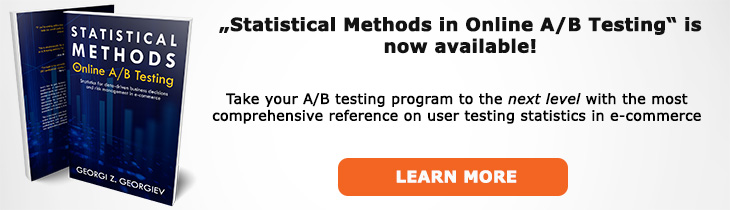# What does "Binomial Metric" mean?

Definition of Binomial Metric in the context of A/B testing (online controlled experiments).

## What is a Binomial Metric?

Aliases: bernoulli metric

A binomial metric, a.k.a. Bernoulli metric is any parameter which can only take two discrete values, usually 0 or 1. This is any variable that measures the presence or absence of something (e.g. conversion, transaction, bounce, unsubscribe event) or measuring something that only has two possible levels or values (e.g. yes or no, male or female). Many of the most used metrics such as conversion rates of all kinds are rates of binomial values (but not binomial themselves!).

A nice property of binomial metrics is that their means (proportions, rates) have known variance and standard deviation: it can be computed analytically and does not require one to estimate it during sample size calculations / power analysis like one would do for a non-binomial metric. Similarly, when calculating statistics for such metrics one does not require the whole data set but only summary statistics such as the proportion and the sample size of each group, making it easier to enter such information into different calculators.

For small sample sizes properties of binomial metrics follow a binomial distribution. For larger sample sizes (large is relative to how close to one end of the distribution the value is) the binomial distribution approximates the normal distribution and the two can be used interchangeably.

## Related A/B Testing terms

Non-Binomial Metric

Like this glossary entry? For an in-depth and comprehensive reading on A/B testing stats, check out the book "Statistical Methods in Online A/B Testing" by the author of this glossary, Georgi Georgiev.## Glossary Index by Letter

Select a letter to see all A/B testing terms starting with that letter or visit the Glossary homepage to see all.